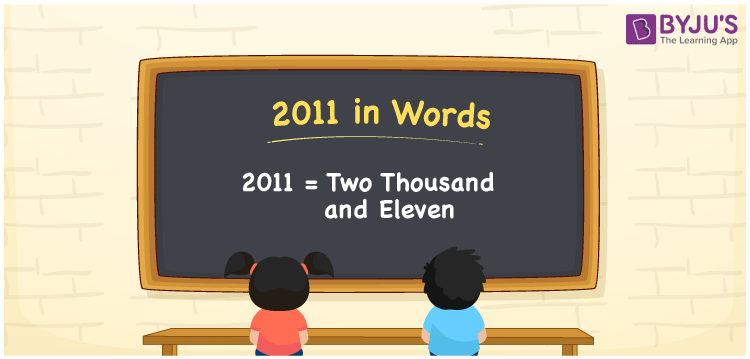# 2011 in Words

Two thousand and eleven is the number name for the number 2011. 2011 is a cardinal number that helps to express the value equivalent to two thousand eleven. For example, the cost of the flower pot is Rs. 2011. Hence, everyone should learn the number in words to express the value. In this article, let us discuss how to write 2011 in words with a complete explanation.

 2011 in Words: Two Thousand and Eleven. Two Thousand and Eleven in Numerical Form: 2011.

## 2011 in English Words## How to Write 2011 in Words?

The place value chart for the four-digit number 2011 is provided below.

 Thousands Hundreds Tens Ones 2 0 1 1

The expanded form of 2011 is as follows:

= 2 × Thousand + 0 × Hundred + 1 × Ten + 1 × One

= 2 × 1000 + 1 × 10 + 1 × 1

= 2000 + 10 + 1

= 2011

= two thousand and eleven

Hence, 2011 in words is two thousand and eleven.

2011 in words – Two thousand and eleven

Is 2011 an odd number? – Yes

Is 2011 an even number? – No

Is 2011 a perfect square number? – No

Is 2011 a perfect cube number? – No

Is 2011 a prime number? – Yes

Is 2011 a composite number? – No

## Frequently Asked Questions on 2011 in Words

Q1

### How to write 2011 in words?

2011 in words is two thousand and eleven.

Q2

### Simplify 2000 + 11, and express it in words.

Simplifying 2000 + 11, we get 2011. Hence, 2011 in words is two thousand and eleven.

Q3

### Is 2011 a prime number?

Yes, 2011 is a prime number.